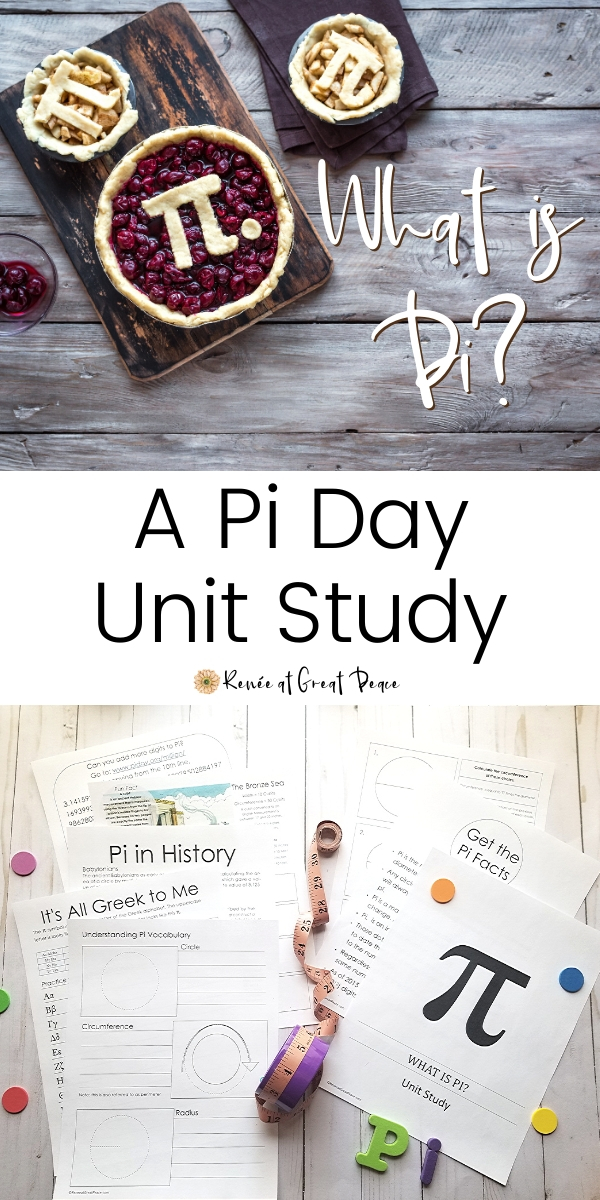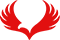World Pi Day is next week! And I’m sharing a mini Pi Day Unit Study with you. Did you know? Or, do you even know what that means?

Pi is the mathematical constant of a circle. Pi is an irrational number, π, which cannot be expressed as a fraction because the decimal equivalent is infinite.

It doesn’t end or show a repeating pattern. Every circle ratio can be calculated to Pi or the approximation of Pi no matter the size of the circle.

## π = C/d

It is equal to the circumference of the circle divided by it’s diameter.

Why is there a World Pi Day? Because the numerical equivalent of Pi is, 3.141592653589… and is almost always shortened to 3.14. Because March the 14th or 3/14 many mathematicians and other geeky folk celebrate the day as World Pi Day.

Interestingly enough, it’s also the birthday of one of the world’s most beloved mathematicians, Albert Einstein.

As homeschoolers, it’s the perfect time to introduce Pi to your would-be mathemascholars. I don’t even like math, in fact, I’m not good at math, at all, but I still think Pi is fun to learn about and explore.

## A Mini Pi Day Unit Study

### Pi Explained

This graphic shows a visual representation of Pi. Imagine, a wheel that has wet paint surrounding the circumference. When it is rolled the paint is left behind. From the center of the wheel to the length of the circumference you can see that Pi is equal to apx. 3.14.

A visual representation of pi. (Image credit: Available at Wikipedia – Author John Reid Creative Commons).

Sal Khan from KhanAcademy.org explains Circles: radius, diameter, circumference and Pi. We use Khan Academy for math in our homeschool. While we often try other programs, time and again, we come back to Khan Academy because of it’s depth, scope and the fact that it teaches the student to the mastery level.

Khan Academy is more than just math videos. It is a complete math curriculum covering basic elementary math through advanced level high school math. In addition, Khan Academy, offers curriculum covering these topics. Science, Technology, Arts and Computing. We also use KA so our young man can learn programming.

### 5 Fun Facts about Pi

1. It’s a mathematical constant.
2. It’s an infinite number (at least no one has ever discovered the last number of pi).
3. It’s an irrational number.
4. The number never changes, it is always. 3.141592653589… (π).
5. It has been calculated to 13.3 Trillion Digits, (1013).

### One Million Digits of Pi

For fun, take a look at this page which shows 1 Million Digits of Pi.

(Because these are Kindle versions you can get them today).

## Study the Music of Pi

Someone even decided to set pi to music. Here you can hear what pi sounds like. Fugue on Pi by Greg Ristow. You can also learn about the Circle of Fifths as part of your World Pi Day music study.

### Pi Math Challenges

Pi is fun to explore. It can be a good challenge to see how many digits a student can memorize.

Here is a Pi Challenge to play which can help to build memorization skills. This one is even more challenging in that it requires a student to think ahead and consider if the next number is higher or lower than the one shown. While this typing test helps a student to build both their typing skills and memorization skills.

Another fun challenge you can do is to have your students collect circles from around the classroom, or the home. Then, using a tape measure, have them measure the diameter of each circle, then measure the circumference of each circle. Have them document each measurement for each circle.

Then, using the mathematical calculation π = C/d have them calculate pi for each circle. So, for each circle, divide circumference by the diameter. Let them use a calculator for this exploration!

### Pi Day Unit Study

I decided to pull together a brief unit study about Pi. Because, well, it’s World Pi Day, it’s fun, and my son loves math! So I’m sharing it with you. It’s free, just click here to download.

World Pi Day is a wonderful way to explore this mathematical phenomenon. Using this free World Pi Day Unit Study will help you to teach all the basics for the day. And, of course, you’ll want to bake up your favorite pie for Pi Day! How fun, to enjoy a pizza pie for dinner, strawberry or apple pie for dessert, all made by the kids for Pi Day life skills.

Don’t Miss these Math Posts

Be sure to Pin It for future reference.

This Post is part of iHomeschool Network’s The Massive Guide to Homeschooling Math.## 2 Replies to “What is Pi? – A Pi Day Unit Study Download”

1.Mother of 3 says:

This is the first year I actually remembered to celebrate Pi day with my kids. We had so much learning about Pi. My 10 year old spent the morning reading the first hundred or so digits in the number out loud.
Mother of 3 recently posted…Pi Day Celebration2. Pingback: Pi Day Resources